### IMO Shortlist 2009 problem N1

Kvaliteta:
Avg: 3,0
Težina:
Avg: 5,5
Let$n$ be a positive integer and let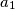$a_1$,$a_2$,$a_3$, ...,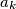$a_k$ (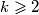$k \geqslant 2$) be distinct integers in the set$\left\{1,\,2,\,\ldots,\,n\right\}$ such that$n$ divides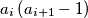$a_i \left(a_{i + 1} - 1\right)$ for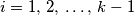$i = 1,\,2,\,\ldots,\,k - 1$. Prove that$n$ does not divide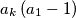$a_k \left(a_1 - 1\right)$.

Proposed by Ross Atkins, Australia
Izvor: Međunarodna matematička olimpijada, shortlist 2009